Rationalize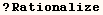The line below finds a rational number that is very close to the machine
number approximation of Pi.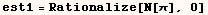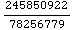Rationalize[N[π],0.0]  gives the same number as the line above.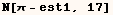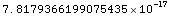The next line gives a rational approximation of π that is remarkably
close to the actual value of π.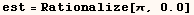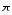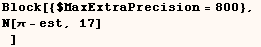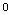If you want to quickly convert a Real to a Rational and you don't care about
large integers in the numerator and denominator the next line should be used.
It's about 26 times faster than Rationalize!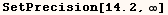Created by Mathematica  (May 16, 2004)

Back to Ted’s Tricks index page